# CBSE Class 10 Maths Chapter 13-Surface Areas and Volumes Objective Questions

Here, in this CBSE Class 10 chapter 13- Surface Areas and Volumes Objective Questions, students will get acquainted with how to solve questions based on surface areas and volumes of different shapes, such as cone, sphere, cylinder and more. Other concepts discussed in this chapter also include the conversion of a solid from one shape to another shape and then finding the surface area and volume of the new shape formed. We also provide the solutions the CBSE Class 10 Maths Chapter 13-Surface Areas and Volumes Objective Questions framed from the chapter of the textbook.

Based on the changed exam pattern that includes more of MCQs, we have covered all the chapter-wise CBSE Class 10 Maths objective questions that are expected to be included in the exam papers for the final exams.

## Sub-Topics Discussed Under Chapter 13

Find here some of the main topics discussed in this chapter.

13.1 Basics (6 MCQs From This Topic)

13.2 Combination of solids (6 MCQs From The Given Topics)

13.3 Shape conversion of solids (7 MCQs Listed From The Topic)

## Download CBSE Class 10 Maths Chapter 13-Surface Areas and Volumes Objective Questions Free PDF

### Basics

1.The lateral surface area of a right circular cone of height 28 cm and base radius 21 cm(in sq. cm) is:

1. 2310
2. 2110
3. 1055
4. 1155

Solution: h = 28 cm; r = 21 cm

Therefore, slant height (i) =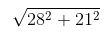= 35 cm

Lateral surface area = πrl

= 22/7 × 21 × 35

= 2310 cm

2.If the ratio of the radius of a cone and a cylinder of equal volume is 3:5, then find the ratio of their heights.

1. 25/3
2. 28/3
3. 23/3
4. 7

Solution: Let r1 and h1 be the radius and height of the cone and r2 and h2 be the radius and height of the cylinder.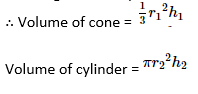It is given that the volume of cone is equal to the volume of cylinder.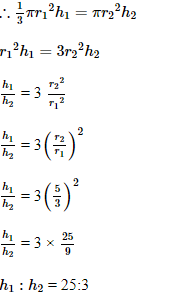Thus, the ratio of their height is 25: 3.

3. An iron rod of diameter 1cm and length 8cm is drawn into a wire of length 18m of uniform thickness. Find the thickness of the wire?

1. 0.09cm
2. 0.08cm
3. 0.06cm
4. 0.05cm

Solution: Volume of the rod = πr2h= (π) × (1/2)2 × 8= 2πcm3

Volume of the wire = πr2h= (π) × (r)2 × 1800 = 1800πr2cm3

Volume of the rod (old solid shape) = Volume of the wire (New solid shape)

2 π=1800πr2

r2=1/900

r = 1/30

, Diameter = 1/15=0.06cm

4. What do you understand by the quantity called ‘area’?

1. It is the height of an object
2. It is the quantity that expresses the extent of a planar 2-D surface
3. It is the length of an object
4. It is the quantity of an object

Answer: (B) It is the quantity that expresses the extent of a planar 2-D surface

Solutions: The first thing that needs to be understood is that area is a 2 dimensional quantity. Area is the quantity that expresses the extent of a two-dimensional figure or shape, or planar lamina, in the plane. It is only possible to measure area for 2-D surfaces. There is a different quantity to deal with areas for 3-D surfaces which we will look at slightly later in the upcoming questions.

Examples of 2-D surfaces are rectangles, circles, ellipse etc. It is possible to find area for all these 2-D surfaces. But area of objects such as cubes, cylinders, spheres etc. are not defined. But intuitively we know that there is some area associated with such 3-D objects. How come? The answer is coming soon!

5. A solid metallic sphere of diameter 21 cm is melted and recast into a number of smaller cones, of diameter 3.5 cm and height 3cm. The number of cones so formed is:

1. 254
2. 504
3. 540
4. 405

Solution: Radius of the sphere= 21/2 cm

Volume of the sphere= (4/3) π (21/2)3cm3

Radius of the cone=7/4 cm and height=3 cm

Volume of cone=1/3πr2h=1/3π (7/4)2×3 cm3

Let the number of cones formed be n. Then,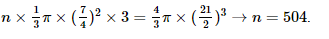6. How many dimensions are required to make a cuboid?

1. 3
2. 1
3. 15
4. 100

### Combination of solids

7.There are 2 identical cubes each having a total surface area equal to ‘A’. Let ‘S’ be the surface area of the solid obtained by joining these 2 cubes end to end. Which of the following statements is true?

1.  Cannot be determined
2. S < 2A
3. S > 2A
4. S = 2A

Solution: When 2 cubes are joined end to end it can be easily figured out that the ends of the 2 cubes which are joined together will not be visible after joining. But all the other faces will remain visible.

The total surface area of combined solid can be obtained by adding surface areas of individual cubes and then subtracting the surface areas of one face of each cube which have become hidden due to joining. The combined solid will be a cuboid whose height and breadth will be same as cube’s side. The length of cuboid will be summation of lengths sides of each cube.

8. The figure consists of 2 cylinders, the inner cylinder is a solid cylinder whose radius is r and the outer cylinder is a hollow cylinder whose radius is R and height is h, the volume of fluid it can hold is: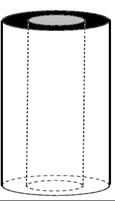1. πr2h
2. πR2h
3. π(R2−r2)h
4. π(R2+r2)h

Solution: The inner cylinder is solid whereas the outer is hollow. If the inner cylinder was not there, then the volume of fluid the outer cylinder can hold would be πR2h but since the inner cylinder is solid and is occupying some space, it is limiting the volume of the outer cylinder.

So, the volume of fluid the given shape can hold is the difference in volume of the outer and inner cylinders.

Volume = π (R2−r2) h

9. Find the volume of the figure.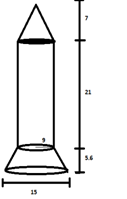1. 3181.2
2. 5162.5
3. 7142.8
4. 8527.2

Solution: Volume of the figure = volume of cone + volume of cylinder + volume of frustum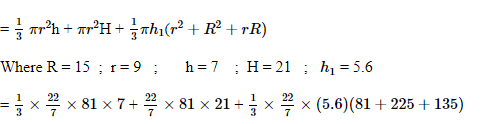= 594 + 5346 + 2587.2

= 8527.2

10. A piece of cloth is required to completely cover a solid object. The solid object is composed of a hemisphere and a cone surmounted on it. If the common radius is 7 m and height of the cone is 1 m, what is the area of cloth required?

1. 262.39m2
2. 463.39m2
3. 662.39m2
4. 563m2

Solution: Surface area of hemisphere = 2πr2

=2×22/7× (7)2 = 308 m2.

For calculating the surface area of a cone we need to calculate its slant height,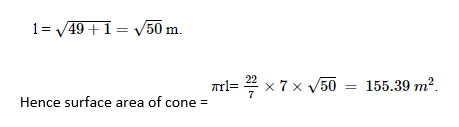So, area of cloth required = (308 + 155.39) m2 = 463.39 m2

11.An oil funnel made of tin sheet consists of a 10 cm long cylindrical portion attached to a frustum of a cone. If the total height is 22 cm, the diameter of the cylindrical portion is 8 cm and diameter of the top of the funnel is 18 cm, find the area of the tin sheet required to make the funnel.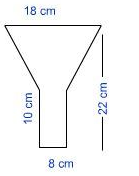1. 525.25 cm2
2. 724.25!cm2
3. 781.86 cm2
4. 700 cm2

Solution: Curved surface area of cylinder

2πrh=π×8×10=80×π

The slant height of frustum can be calculated as follows: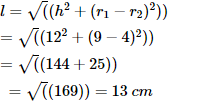Curved surface area of frustum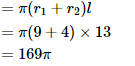Total curved surface area

=169π+80π

=249×3.14

=781.86 cm2

12.Ram has a semicircular disc. He rotates it about its diameter by 360 degrees. When he rotates the disc, a volume of air in his room gets swept. What is the name of the object/shape that exactly occupies this volume?

1. Cylinder
2. Hemisphere
3. Sphere
4. Cuboid

Solution: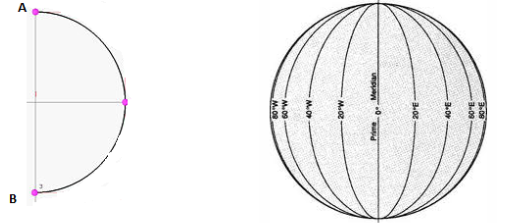It is clear that by seeing 2nd diagram we can know how semicircle sweeps and what is the shape obtained. The shape obtained is a sphere. If rotation had been done for only 180 degrees instead of 360 degrees, we get a hemisphere.  The line segment AB which acted as axis of rotation will also be diameter of sphere formed.

### Shape conversion of solids

13.A bucket is in the form of a frustum of a cone, its depth is 15 cm and the diameters of the top and the bottom are 56 cm and 42 cm respectively. How many liters of water can the bucket hold?

1. 28.49
2. 7.5
3. 2.5
4. 10

Solution: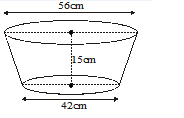R = 28 cm

r = 21 cm

h = 15 cm

Capacity of the bucket = 1/3πh (R2+r2+Rr)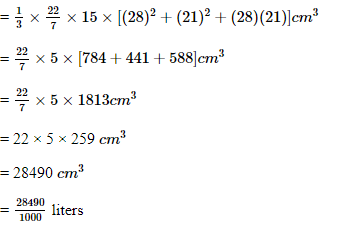= 28.49 liters

14. A 20 m deep well of diameter 7 m is dug and the earth taken out is evenly spread out to form a platform of 22 m by 14 m. Find the height of the platform (in m).

1. 7.5
2. 2.5
3. 10
4. 5

Solution: Diameter of the well = d = 7 m

⇒ Radius of the well = r = 7/2=3.5m

Height of the well = h =20 m

Length of the platform = 1 = 22 m

Breadth of the platform = b = 14 m

Let height of the platform =h

According to given condition we have:

Volume of Well = Volume of Earth Dug out

⇒ π. r2.h= l × b × h1

⇒22/7 × 3.5 × 3.5 × 20=22×14×h1

⇒h1=22/7 × 3.5 × 3.5 × 20 × 122 × 114

⇒h1=2.5m

15. A cylindrical tank is filled by pumping water from a cuboidal tank of dimensions 200cm × 150cm × 95 cm. The radius of the cylindrical tank is 60cm and height is 95cm. Find the height (in m) of the water left in the cuboidal tank after the cylindrical tank is completely filled. (Take π = 3.14)

1. 0.76 m
2. 0.69 m
3. 0.59 m
4. 0.45 m

Solution: Volume of the cylindrical tank = πr2h= (3.14) × (0.6)2×0.95m=1.07m3

Volume of the Cuboidal tank when full = l × b × h = (2m × 1.5m × 0.95m) = 2.85 m3

Volume of water left in the cuboidal tank after completely filling the cylindrical tank = 2.85 – 1.07) = 1.78 m3

Height of water left in cuboidal tank = Volume of water left in cuboidal tank / (l × b)

= 1.78/ (2 × 1.5) = 0.59m

16. A cylinder is moulded into the shape of a sphere. Which of the following factors will be same for both the shapes?

1. None of these
2. Curved surface area
3. Surface area
4. Volume

Solution: Volume is a factor which does not differ with change of shape. A cylinder can be moulded into a sphere or a cube or a cuboid of varying dimensions keeping the volume constant.

17. Water in a canal, 6 m wide and 1.5 m deep, is flowing with a speed of 10 km/h. How much area will it irrigate (in m2) in 30 minutes, if 8 cm of standing water is needed?

1. 256500
2. 526500
3. 625500
4. 562500

Solution: Speed of water flowing through canal =10 km/h =10, 000 m/h

Volume of water flowing through canal in 1 hour = 6 × 1.5 × 10,000 = 90,000 m3

Volume of water flowing through canal in 30 minutes = 90000/2=45,000m3

Standing water = 8 cm =0.08 m

According to given condition we have:

Area which can be irrigated × 0.08 = 45000

⇒ Area which can be irrigated = 45000/ 0.08=562500m2

18. The diameter of a sphere is 6 cm. It is melted and drawn into a wire of diameter 2 mm. The length of the wire is:

1. 66m
2. 36m
3. 18m
4. 12m

Solution: Diameter of metallic sphere=6 cm

∴ Radius of metallic sphere=3 cm

Also, we have diameter of cross-section of cylindrical wire=0.2 cm

∴ Radius of cross-section of cylindrical wire=0.1 cm

Let the length of the wire be h cm

Since metallic sphere is converted into a cylinder shaped wire of length h cm

∴ Volume of the metal used in wire = Volume of the sphere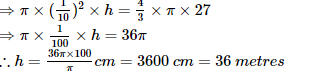19. How many gold coins of 1.75cm in diameter and 2mm in thickness can be melted to form a cuboid of dimensions 5.5cm x 10cm x 3.5cm?

1. 400
2. 500
3. 350
4. 550

Solution: Radius of the coin = 0.875cm

Height of coin = 0.2 cm

Volume of the cylinder =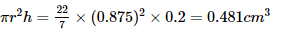Volume of cuboid = l × b × h = 5.5 × 10 × 3.5

= 192.5 cm3

Let x be the number of coins that can be made

So, x = volume of cuboid / volume of the cylinder (coin) =192.5/ 0.481= 400 coins

CBSE Class 10 Maths Chapter 13-Surface Areas and Volumes Objective Questions are made available for the students who want to excel in the board exam. With the help of these solved questions, students can get practice and thus prepare well for the board exams.

There are also some extra questions from CBSE Class 10 Maths Chapter 13 here, which the students can solve for practice.

### CBSE Class 10 Maths Chapter 13 Extra Questions

1. What is a cylindrical pencil sharpened at one edge the combination of?
(a) a cone and a cylinder
(b) a hemisphere and a cylinder
(c) two cylinders
(d) frustum of a cone and a cylinder

2. Given that a right circular cylinder is of radius r cm and height h cm (h>2r), _______ encloses a sphere of diameter
(a) 2r cm
(b) r cm
(c) 2h cm
(d) h cm

3.  When twelve solid spheres of the same size are made by melting a solid metallic cylinder of base diameter 2 cm and height 16 cm. Then, calculate the diameter of each sphere.
(a) 4 cm
(b) 6 cm
(c) 3 cm
(d) 2 cm

Keep learning and stay tuned to get the latest updates on CBSE along with syllabus, marking scheme, sample papers, previous year question papers and more.